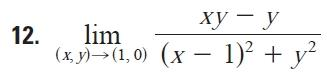# Show the limit exists or does not exist

• vantz

## Homework Statement## The Attempt at a Solution

it does not exist, but i can't get different limits, I am only getting it approaches 0

i replaced x=1 only, y=0 only, y=x, x=y but I'm only getting 0 as my limit.

thanks

Last edited:

this is a trick. notice it is equivalent to finding the limit of [xy]/(x^2+y^2) as (x,y)-->(0,0).

this one is very familiar, and is done by letting x=y.

im still approaching 0 when x=y

im still approaching 0 when x=y

You shouldn't be. Replacing y with x:

$$\lim_{(x,y) \to (0,0)} \frac{x*x}{x^2+x^2} = ...$$

oh so first i rewrite the limit into a more familiar one and then solve. but i don't see how they are related.

edit:nvm i got it.

Last edited:

## Homework Statement## The Attempt at a Solution

it does not exist, but i can't get different limits, I am only getting it approaches 0

i replaced x=1 only, y=0 only, y=x, x=y but I'm only getting 0 as my limit.

thanks
Yes, if you let x=1, and take the limit of the result as y→0, the limit is zero.

Similarly, letting y=0 and taking the limit of the result as x→1, also gives zero.

Use these results along with the limit obtained as others have suggested. What's your final conclusion?

Yes, if you let x=1, and take the limit of the result as y→0, the limit is zero.

Similarly, letting y=0 and taking the limit of the result as x→1, also gives zero.

Use these results along with the limit obtained as others have suggested. What's your final conclusion?

i showed the relation between the original limit and [xy]/(x^2+y^2) as (x,y)-->(0,0).
numerator factorizes to y(x-1), then i replaced (x-1) with t, and as x→1, t→0. new limit does not exist because it approaches 1/2 and 0.

Correct !